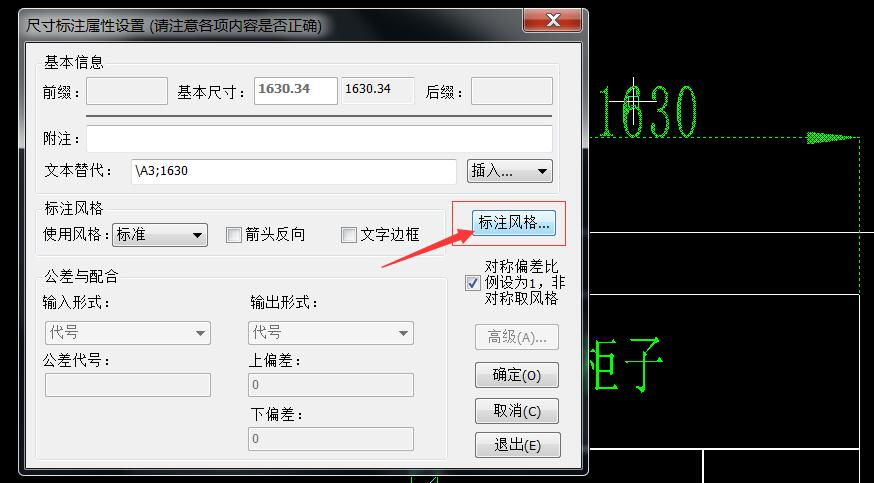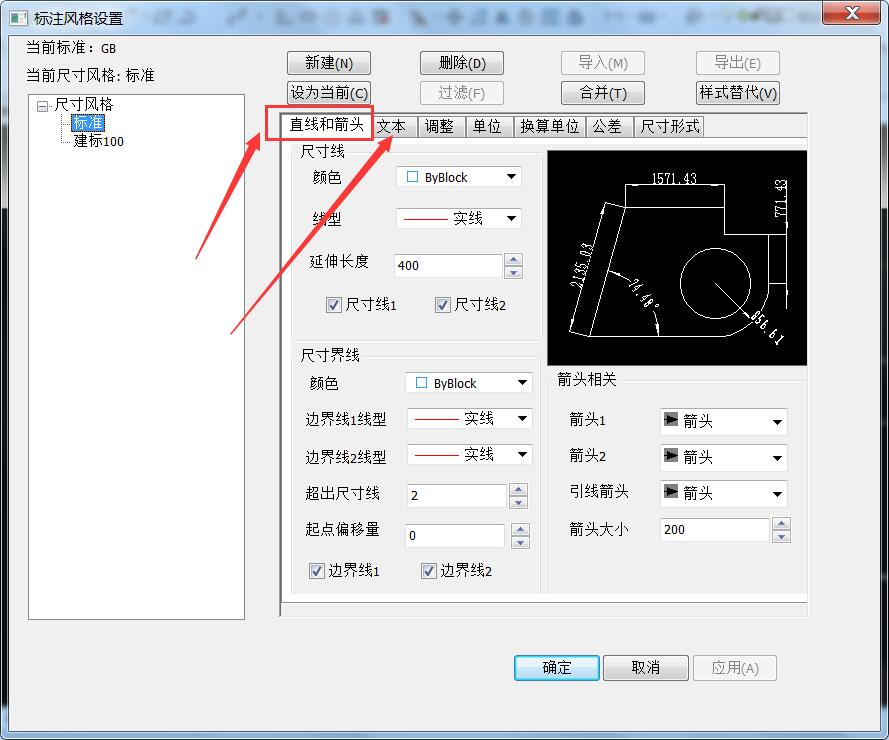• 说明 此博客为任务驱动而记录，在一次画图...‘操作’，‘数量’，‘价格’：在上一要求画出的折线图中标注对应点，比如 时刻 ‘2020/8/1 9:37’ 处在折线图对应点上标注出 ‘操作’、‘数量’、‘价格’ 信息；并且
说明
此博客为任务驱动而记录，在一次画图工作中，总结了一些画图技巧，遂记录下来便于日后画图时有所参照，如果你也遇到一些画图上的问题，希望这篇博客有可能帮到你
任务详情
数据
example_csdn.csv需要对该文件进行绘图，要求是：

‘time’，‘data’：以‘time’列为横坐标，绘制‘data’列的折线图；
‘操作’，‘数量’，‘价格’：在上一要求画出的折线图中标注对应点，比如时刻‘2020/8/1 9:37’处在折线图对应点上标注出‘操作’、‘数量’、‘价格’信息；并且‘操作’中对‘买’、‘卖’标注出不同颜色。

操作
数据预处理
折线图好画，但是在某些点上进行分类标注，一时没想到什么简便方法，所以用一个笨法子，做一个预处理，根据‘data’列、‘操作’列生成新的两列：第一列为‘buy’，‘操作’列中为‘买’的时刻，‘buy’列对应时刻设置为‘data’列对应时刻的值，其他位置为空值；第二列为‘sell’，‘操作’列中为‘卖’的时刻，‘sell’列对应时刻设置为‘data’列对应时刻的值，其他位置为空值。如下图示程序比较简单，如下：
def preprocess(ori_file, save_file):
df = pd.DataFrame(pd.read_csv(ori_file, encoding='gbk'))
df = df.set_index(pd.DatetimeIndex(pd.to_datetime(df.time)))
df.drop(columns=['time'], inplace=True)

print(df[:20])
print(df.shape)
print(df.columns)

buy_list = []
sell_list = []
data_list = df['data']
operation_list = df['操作']
for i in range(df.shape):
if operation_list[i] == '0':
buy_list.append(np.nan)
sell_list.append(np.nan)
if operation_list[i] == "买":
buy_list.append(data_list[i])
sell_list.append(np.nan)
if operation_list[i] == "卖":
buy_list.append(np.nan)
sell_list.append(data_list[i])

df['buy'] = buy_list
df['sell'] = sell_list

print(df[:20])
print(df.shape)
print(df.columns)

df.to_csv(save_file, index=True, encoding='gbk')

做了数据预处理后，任务需求大概框架就可以是
依据‘data’列绘制折线图，在同一幅图中，使用相同横坐标依据‘buy’列、‘sell’列绘制散点图，对散点图上的点加标注信息。
画图
折线图
fig, ax = plt.subplots(1, 1)
# ---------- 标题 ----------
title = "示例 " + name
# fontsize: 字体大小
plt.title(title, fontsize=16)

# ---------- 折线图 ----------
# x 为横坐标 'time' 列
# y1 为 'data' 列
# color: 颜色
# lw: 线宽
ax.plot(x, y1, color='royalblue', lw=2.5, label='数据')
# 不加下面这句 label 显示不出来
plt.legend()

# 折线图中 label 的字体大小
font = {'size': 14}
matplotlib.rc('font', **font)

散点图
# ---------- 散点图 ----------
# x 为横坐标 'time' 列
# y2 为 'buy' 列
# y3 为 'sell' 列
# marker: 点的形状
# c: 颜色
ax.scatter(x, y2, marker='o', c='darkgreen')
ax.scatter(x, y3, marker='o', c='firebrick')

图中标注文字信息
# ---------- 标注 ----------
# ----- 生成要显示的信息 -----
# 操作为 '买' 时要显示的信息
text_buy = []
for i in range(len(x)):
if (df['操作'][i] != 0) and (df['操作'][i] == "买"):
str_info = '价格: ' + str(df['价格'][i]) + '\n' + '数量: ' + str(df['数量'][i]) + '\n' + '操作: ' + str(df['操作'][i])
text_buy.append(str_info)
else:
text_buy.append(" ")
# 操作为 '卖' 时要显示的信息
text_sell = []
for i in range(len(x)):
if (df['操作'][i] != 0) and (df['操作'][i] == "卖"):
str_info = '价格: ' + str(df['价格'][i]) + '\n' + '数量: ' + str(df['数量'][i]) + '\n' + '操作: ' + str(df['操作'][i])
text_sell.append(str_info)
else:
text_sell.append(" ")

# ----- 买 -----
# 标注框
# boxstyle: 标注框形式
# fc: 标注框背景颜色
# alpha: 标注框背景透明度
bbox_buy = dict(boxstyle="round", fc='lightgreen', alpha=0.6)
# 标注箭头
# arrowstyle: 箭头形式
# connectionstyle: 连线形式
arrowprops_buy = dict(arrowstyle="->", connectionstyle="arc3,rad=0.")

# 标注框偏移量
# x 轴偏移量
offset_x = 60
# y 轴偏移量
offset_y = 60

# 交替标志
# 此标志的作用是防止两个标注信息点离得过近导致标注信息重叠
# 相邻的点存在标注信息时，通过不同的交替标志绘制不同方向的标注信息
flag = 0

# 对列循环进行标注
for i in range(len(x)):
if text_buy[i] != " ":
if flag == 0:
flag = 1
# text_buy[i] 为要显示的标注信息列表，注意要与横坐标同尺寸
# xy: 标注信息的位置，(横坐标, 纵坐标)
# xytext: 标注信息相对于标注信息位置的偏移量，由 offset_x 和 offset_y 限定
# textcoords: 配合 xytext 使用，具体含义未研究，sorry，可取 'offset points' or 'offset pixels'
# bbox: 标注框设置
# arrowprops: 标注设置
# size: 标注框大小，标注框里的信息会随框大小变化而变化
plt.annotate(text_buy[i],
xy=(x[i], y2[i]),
xytext=(-offset_x * 4, offset_y * 0.9),
textcoords='offset points',
bbox=bbox_buy,
arrowprops=arrowprops_buy,
size=15)
else:
flag = 0
plt.annotate(text_buy[i],
xy=(x[i], y2[i]),
xytext=(offset_x, -offset_y * 0.9),
textcoords='offset points',
bbox=bbox_buy,
arrowprops=arrowprops_buy,
size=15)
else:
plt.annotate(text_buy[i],
xy=(x[i], y2[i]),
xytext=(offset_x, -offset_y * 0.9 / 2),
textcoords='offset points',
bbox=bbox_buy,
arrowprops=arrowprops_buy,
size=15)

# ----- 卖 -----
# 标注框
bbox_sell = dict(boxstyle="round", fc='salmon', alpha=0.6)
# 标注箭头
arrowprops_sell = dict(arrowstyle="->", connectionstyle="arc3,rad=0.")

# 交替标志
flag = 0

# 对列循环进行标注
for i in range(len(x)):
if text_sell[i] != " ":
if flag == 0:
flag = 1
plt.annotate(text_sell[i],
xy=(x[i], y3[i]),
xytext=(-offset_x * 4, offset_y * 0.9),
textcoords='offset points',
bbox=bbox_sell,
arrowprops=arrowprops_sell,
size=15)
else:
flag = 0
plt.annotate(text_sell[i],
xy=(x[i], y3[i]),
xytext=(offset_x, -offset_y * 0.9),
textcoords='offset points',
bbox=bbox_sell,
arrowprops=arrowprops_sell,
size=15)
else:
plt.annotate(text_sell[i],
xy=(x[i], y3[i]),
xytext=(offset_x, -offset_y * 0.9 / 2),
textcoords='offset points',
bbox=bbox_sell,
arrowprops=arrowprops_sell,
size=15)

设置横坐标密度，调整横坐标旋转角度
由于横坐标为时间，并且数据列比较长，如果不做处理的话横坐标无法正常显示，类似下图情况、这里对这种密集的时间横坐标做两个操作

调整图中横坐标显示密度，或者说对‘time’列进行采样后再显示
由于横坐标一个刻度显示内容过多，刻度间间隔过小，所以调整横坐标刻度值显示方向：旋转90°显示

# ---------- 坐标轴 ----------
# 设置 x 轴采样大小
tick_spacing = 10
ax.xaxis.set_major_locator(ticker.MultipleLocator(tick_spacing))
# 设置 x 坐标轴标签的显示内容和大小
plt.xlabel('时间', fontsize=14)
# 设置 x 坐标轴刻度的旋转方向和大小
# rotation: 旋转方向
plt.xticks(rotation=90, fontsize=14)
# 设置 y 坐标轴刻度大小
plt.yticks(fontsize=14)

让内容处于整幅图中合适的位置
# 调整图的位置
plt.subplots_adjust(top=0.9, bottom=0.2, left=0.15, right=0.95)

保存设置
# 设置保存尺寸
fig.set_size_inches(28, 20)
# 设置保存清晰度，并保存图像
plt.savefig(save_picture, dpi=300)

结果完整程序
import numpy as np
import pandas as pd
import matplotlib
import matplotlib.pyplot as plt
import matplotlib.ticker as ticker

def preprocess(ori_file, save_file):
df = pd.DataFrame(pd.read_csv(ori_file, encoding='gbk'))
df = df.set_index(pd.DatetimeIndex(pd.to_datetime(df.time)))
df.drop(columns=['time'], inplace=True)

print(df[:20])
print(df.shape)
print(df.columns)

buy_list = []
sell_list = []
data_list = df['data']
operation_list = df['操作']
for i in range(df.shape):
if operation_list[i] == '0':
buy_list.append(np.nan)
sell_list.append(np.nan)
if operation_list[i] == "买":
buy_list.append(data_list[i])
sell_list.append(np.nan)
if operation_list[i] == "卖":
buy_list.append(np.nan)
sell_list.append(data_list[i])

df['buy'] = buy_list
df['sell'] = sell_list

print(df[:20])
print(df.shape)
print(df.columns)

df.to_csv(save_file, index=True, encoding='gbk')

def draw_picture(save_file, name, save_picture):

df = pd.DataFrame(pd.read_csv(save_file, encoding='gbk'))

x = df['time']
y1 = df['data']

y2 = df["buy"]
y3 = df["sell"]

fig, ax = plt.subplots(1, 1)
# ---------- 标题 ----------
title = "示例 " + name
# fontsize: 字体大小
plt.title(title, fontsize=16)

# ---------- 折线图 ----------
# color: 颜色
# lw: 线宽
ax.plot(x, y1, color='royalblue', lw=2.5, label='data')
# 不加下面这句 label 显示不出来
plt.legend()

# 折线图中 label 的字体大小
font = {'size': 14}
matplotlib.rc('font', **font)

# ---------- 散点图 ----------
# marker: 点的形状
# c: 颜色
ax.scatter(x, y2, marker='o', c='darkgreen')
ax.scatter(x, y3, marker='o', c='firebrick')

# ---------- 标注 ----------
# ----- 生成要显示的信息 -----
# 操作为 '买' 时要显示的信息
text_buy = []
for i in range(len(x)):
if (df['操作'][i] != 0) and (df['操作'][i] == "买"):
str_info = '价格: ' + str(df['价格'][i]) + '\n' + '数量: ' + str(df['数量'][i]) + '\n' + '操作: ' + str(df['操作'][i])
text_buy.append(str_info)
else:
text_buy.append(" ")
# 操作为 '卖' 时要显示的信息
text_sell = []
for i in range(len(x)):
if (df['操作'][i] != 0) and (df['操作'][i] == "卖"):
str_info = '价格: ' + str(df['价格'][i]) + '\n' + '数量: ' + str(df['数量'][i]) + '\n' + '操作: ' + str(df['操作'][i])
text_sell.append(str_info)
else:
text_sell.append(" ")

# ----- 买 -----
# 标注框
# boxstyle: 标注框形式
# fc: 标注框背景颜色
# alpha: 标注框背景透明度
bbox_buy = dict(boxstyle="round", fc='lightgreen', alpha=0.6)
# 标注箭头
# arrowstyle: 箭头形式
# connectionstyle: 连线形式
arrowprops_buy = dict(arrowstyle="->", connectionstyle="arc3,rad=0.")

# 标注框偏移量
# x 轴偏移量
offset_x = 60
# y 轴偏移量
offset_y = 60

# 交替标志
# 此标志的作用是防止两个标注信息点离得过近导致标注信息重叠
# 相邻的点存在标注信息时，通过不同的交替标志绘制不同方向的标注信息
flag = 0

# 对列循环进行标注
for i in range(len(x)):
if text_buy[i] != " ":
if flag == 0:
flag = 1
# text_buy[i] 为要显示的标注信息列表，注意要与横坐标同尺寸
# xy: 标注信息的位置，(横坐标, 纵坐标)
# xytext: 标注信息相对于标注信息位置的偏移量，由 offset_x 和 offset_y 限定
# textcoords: 配合 xytext 使用，具体含义未研究，sorry，可取 'offset points' or 'offset pixels'
# bbox: 标注框设置
# arrowprops: 标注设置
# size: 标注框大小，标注框里的信息会随框大小变化而变化
plt.annotate(text_buy[i],
xy=(x[i], y2[i]),
xytext=(-offset_x * 4, offset_y * 0.9),
textcoords='offset points',
bbox=bbox_buy,
arrowprops=arrowprops_buy,
size=15)
else:
flag = 0
plt.annotate(text_buy[i],
xy=(x[i], y2[i]),
xytext=(offset_x, -offset_y * 0.9),
textcoords='offset points',
bbox=bbox_buy,
arrowprops=arrowprops_buy,
size=15)
else:
plt.annotate(text_buy[i],
xy=(x[i], y2[i]),
xytext=(offset_x, -offset_y * 0.9 / 2),
textcoords='offset points',
bbox=bbox_buy,
arrowprops=arrowprops_buy,
size=15)

# ----- 卖 -----
# 标注框
bbox_sell = dict(boxstyle="round", fc='salmon', alpha=0.6)
# 标注箭头
arrowprops_sell = dict(arrowstyle="->", connectionstyle="arc3,rad=0.")

# 交替标志
flag = 0

# 对列循环进行标注
for i in range(len(x)):
if text_sell[i] != " ":
if flag == 0:
flag = 1
plt.annotate(text_sell[i],
xy=(x[i], y3[i]),
xytext=(-offset_x * 4, offset_y * 0.9),
textcoords='offset points',
bbox=bbox_sell,
arrowprops=arrowprops_sell,
size=15)
else:
flag = 0
plt.annotate(text_sell[i],
xy=(x[i], y3[i]),
xytext=(offset_x, -offset_y * 0.9),
textcoords='offset points',
bbox=bbox_sell,
arrowprops=arrowprops_sell,
size=15)
else:
plt.annotate(text_sell[i],
xy=(x[i], y3[i]),
xytext=(offset_x, -offset_y * 0.9 / 2),
textcoords='offset points',
bbox=bbox_sell,
arrowprops=arrowprops_sell,
size=15)

# ---------- 坐标轴 ----------
# 设置 x 轴显示密度
tick_spacing = 10
ax.xaxis.set_major_locator(ticker.MultipleLocator(tick_spacing))
# 设置 x 坐标轴标签的显示内容和大小
plt.xlabel('时间', fontsize=14)
# 设置 x 坐标轴刻度的旋转方向和大小
plt.xticks(rotation=90, fontsize=14)
# 设置 y 坐标轴刻度大小
plt.yticks(fontsize=14)

# 坐标轴中文显示
plt.rcParams['font.sans-serif'] = ['SimHei']

# # x 坐标轴显示完整
# plt.tight_layout()  # 此例中使用标注导致此条语句不能使用

# 调整图的位置
plt.subplots_adjust(top=0.9, bottom=0.2, left=0.15, right=0.95)

# 设置保存尺寸
fig.set_size_inches(28, 20)
# 设置保存清晰度，并保存图像
plt.savefig(save_picture, dpi=300)

plt.show()

if __name__ == "__main__":
ori_file = './example_csdn.csv'
save_file = './example_csdn_processed.csv'
save_picture = './examples_csdn.jpg'
name = 'example'

preprocess(ori_file, save_file)
draw_picture(save_file, name, save_picture)


附录
颜色设置：参考

（原）python中matplotlib的颜色及线条控制
matplotlib命令与格式：设置折线与点属性
如何使pylab.savefig（）将图像保存为“最大化”窗口而不是默认大小
在matplotlib绘图，如何更改字体大小


展开全文python matplotlib
• 设置标注字体、箭头大小  1.双击文字或箭头后 2. 然后
设置标注字体、箭头大小
1.双击文字或箭头后2. 然后展开全文• AutoCAD中标注样式的设置规范（自我总结）1、线---标注规范2、符号和箭头---标注规范3、文字---标注规范4、调整---标注规范5、主单位---标注规范 ...文字高度为文字大小，可根据实际情况进行设置，一般为2.5~3； 从
AutoCAD中标注样式的设置规范（自我总结）1、线---标注规范2、符号和箭头---标注规范3、文字---标注规范4、调整---标注规范5、主单位---标注规范

该标注规范以ISO-25为基准规范样式进行修改

1、线—标注规范

超出尺寸线设置为2；
起点偏移量设置为3；
勾选固定长度的尺寸界线并设置为3；
颜色按照自己喜好进行设置；2、符号和箭头—标注规范

箭头选择建筑标记；
箭头大小调整为0.8；3、文字—标注规范

文字高度为文字大小，可根据实际情况进行设置，一般为2.5~3；
从尺寸线便宜调整为0.1；
文字对齐勾选ISO标准；4、调整—标注规范

从尺寸界线中移出勾选：文字始终保持在界线之间；
文字位置勾选尺寸线上方，不带引线；5、主单位—标注规范

精度一般设置为0；个别情况需要设置为0.0；
比例因子根据图形大小进行设置；展开全文• 我们一般在拿到一张图纸，在看图软件中打开之后，图案错综复杂，我们区分不了，那这个时候，就需要进行文字标注了，那怎么进行标注文字呢？小编为你演示方法。 第一步：在你自己的电脑做桌面上打开一款专业的CAD看...
我们一般在拿到一张图纸，在看图软件中打开之后，图案错综复杂，我们区分不了，那这个时候，就需要进行文字标注了，那怎么进行标注文字呢？小编为你演示方法。
第一步：在你自己的电脑做桌面上打开一款专业的CAD看图软件，还有需要进行标注的图纸文件。

第二步：打开该运用软件，在上方的菜单栏里面打开需要标注的图纸文件。

第三步：图纸打开之后，我们可运用鼠标，再找到左边工具栏中的【平移】设置，进行相应的大小调整，以便于下一步的操作。

第四步：在左侧的属性框中找到【标注】，直接在需要标注的地方单击鼠标左键，在弹出的文字选矿中输入相应的文字即可。

第五步：完成编辑之后，在上方的工具栏中点击【另存为】就可直接保存下来了。

步骤很简单，我们只需要进行相应的标注就可以了，还可以进行其他的编辑工作。
展开全文CAD图纸
• 插件提供50个呼出指示线标注文字标题介绍动画 ，带出入动画，文字支持中文和英文输入，可修改文字字体，位置，颜色，大小，以及线条线框的颜色大小等。可插入文字标题，LOGO，图片，数字等。线条和标注点均可设置...
• 在浩辰CAD看图王电脑版中，可以设置标注的比例、单位、精度、颜色、线宽、文字高度以及箭头大小等等，帮助我们设置不同的标注样式，如下图中三种不同的标注标注位置：浩辰CAD看图王电脑版—编辑模式—测量标注—...
• 在浩辰CAD看图王电脑版中，可以设置标注的比例、单位、精度、颜色、线宽、文字高度以及箭头大小等等，帮助我们设置不同的标注样式，如下图中三种不同的标注标注位置：浩辰CAD看图王电脑版—编辑模式—测量标注—...
• 在设计图纸的时候，我们会将图纸里面的一些文字进行放大标注，如果说文字设置的不合适，我们就需要去调节文字的字体大小，那么我们需要怎么做，才能将CAD文件里面的文字大小进行修改呢？我们接着往下看。 1，下载...
• fontsize设置字体大小，默认12，可选参数 ['xx-small', 'x-small', 'small', 'medium', 'large','x-large', 'xx-large'] fontweight设置字体粗细，可选参数 ['light', 'normal', 'medium', 'semibold', 'matplotlib python 标题
• 此篇为 一次python画图经历——涉及 matplotlib 的折线图，散点图，图中标注文字信息，设置横坐标密度，调整横坐标旋转角度，文字大小、各部分颜色、保存形式、美化调整 的延续，当时自以为很好地处理了标注信息的...python matplotlib
• 尺寸标注样式是决定尺寸标注外观的一组设置，例如，可以通过属性设置一组尺寸标注的尺寸线的颜色、箭头大小标注文字高度，然后为这些尺寸创建一个尺寸标注样式，以后标注尺寸时，只要将这一尺寸标注样式设置为当前...
• fontsize设置字体大小，默认12，可选参数 [‘xx-small’, ‘x-small’, ‘small’, ‘medium’, ‘large’,‘x-large’, ‘xx-large’] fontweight设置字体粗细，可选参数 [‘light’, ‘normal’, ‘medium’, ...
• 右键图层名，【属性】-【标注】-字体大小设置为1，点击【符号】-【编辑符号】-【掩膜】可以设置文字阴影和边框 文字注记换行和拼串： 以VBScript为例，表达式子应为[point_code] & vbnewline & [point_...
• 2.3 标注 ...Maplex是ArcGIS的高级标注引擎，它提供了一系列的工具用于提高地图的标注质量，如控制标注大小和位置、优化标注放置的位置、制定不同要素层标注的重要级别实行压盖避让、控制文字间距、删除
• fontsize设置字体大小，默认12，可选参数 [‘xx-small’, ‘x-small’, ‘small’, ‘medium’, ‘large’,‘x-large’, ‘xx-large’] fontweight设置字体粗细，可选参数 [‘light’, ‘normal’, ‘medium’, ...数据可视化 Matplotlib
• TEECHART使用Canvas.TextOut标注文字，然后我想把TEECHART和标注的文字一起保存成图片（CHART1.SaveToBitmapFile('D:\6789.BMP')）。但是发现保存了，那个标注的文字没有了。图表曲线倒是在的，怎么解决的？ 解决...
• 具体功能：首先设置行进速度，然后在地图上添加点图标注作为起点，从起点绘制路线，在路线上添加中途点文字说明。按“开始演示”后，起点的点图标注将沿着路线运动，当经过路线上的中途点时，有对应的文字说明弹出。...
• 我们都知道文字一般用sp，很少用xp，然而一旦用sp标注文字的大小当系统的文字大小切换时，app文字也跟着切换会导致布局问题，因此为保持文字不变，可以在baseActivty 中重写一下方法，它会在onCreat 调用，重新初始...Activity 字体
• Word 资料 7.1 第1题 操作目的灯管字效果制作要求完成的最后效果如下 操作要求 1建一个新文件1612cm72像素/英寸RGB模式 2制作欢迎文字文字字体任选文字大小为160像素 3将文字制作成灯管字效果将最后结果以文件Xps8-......